# Check Library¶

## Introduction¶

The check library is an assertion library for VHDL providing the more commonly used assertions as a number of check procedures and functions.

## Architecture¶

The check subprograms are conditional log messages. If the condition represents a failing check then an error message is issued using the VUnit log package.

Every check you do is handled by a checker. There is a default checker that is used when none is specified but you can also create multiple custom checkers. For example

```check(re = '1', "Expected active read enable at this point");
```

will use the default checker while

```check(my_checker, re = '1', "Expected active read enable at this point");
```

will use the custom `my_checker`. A custom checker is just a variable or constant initialized with the new_checker function.

```constant my_checker : checker_t := new_checker("my_checker");
```

All subprograms presented in this user guide are available for both the default checker and custom checkers. The difference is the first `checker` parameter which only exists for custom checker subprograms. To make the user guide more compact we present this as an optional parameter using brackets. For example

```impure function check_false(
[constant checker   : in checker_t;]
constant expr      : in boolean;
constant msg       : in string      := result(".");
constant level     : in log_level_t := null_log_level;
constant line_num  : in natural     := 0;
constant file_name : in string      := "")
return boolean;
```

The full verbose API description can always be found in check_api.vhd.

## Checker Creation¶

Since a check is a conditional log it uses a `logger_t` from the logging library described in the logging user guide under the hood.

A `checker_t` is created with a logger name used for the logger internally. Additionally there is a `default_log_level` parameter to specify the log level of a failing check.

```impure function new_checker (
constant logger_name : in string;
constant default_log_level  : in log_level_t  := error)
return checker_t;
```

## Check¶

The check library provides a basic `check` procedure which is similar to the VHDL `assert` statement

```check(re = '1', "Expected active read enable at this point");
```

The first parameter is the boolean expression to check and the second parameter is the error message issued if the expression is false. Assuming this check fails and you haven’t changed the default settings for the default checker the error message will be something like

```10000 ps - check -  ERROR - Expected active read enable at this point
```

If you wish to have another log level than the default one set by `new_checker` you can override this for each check call. For example

```check(re = '1', "Expected active read enable at this point", failure);
```

A failing check is always counted as a failing check regardless of the level but the level affects whether or not the simulation stops as controlled by the stop level for the internal logger. The stop level can be change by retrieving the logger and then use the `set_stop_level` procedure as described in logging user guide. For example

```set_stop_level(get_logger(my_checker), warning);
```

Note that when using the VUnit Python test runner the default checker stop level is set to `error` when calling `test_runner_setup`. The reason is that the Python test runner has the ability to restart the simulation with the next test case so that all test cases are run despite of an error while guaranteeing that the error state of the failing test case doesn’t propagate into the next. If you’re not using the Python test runner the stop level is set to `failure` as a way to continue on `error` but without this guarantee.

### Logging Passing Checks¶

The provided message in a check call is also logged when the check passes. The typical use case is to create a debug trace to investigate what happened before a bug occured. This feature uses the log level `pass` that is not visible by default but can be enabled for any log handler.

```show(get_logger(default_checker), display_handler, pass);
```

The difference between a passing check log message and a failing check log message is the log level used. A passing check like this

```check(re = '1', "Checking that read enable is active");
```

will result in a log entry like this

```1000 ps - check - PASS - Checking that read enable is active
```

Note that a message that reads well for both the pass and the fail cases was used.

A number of check subprograms perform several checks for every call, each of which can fail and generate an error message. However, there will only be one pass message for such a call to avoid confusion. For example, `check_stable` checks the stability of a signal for every clock cycle in a window. If the window is 100 clock cycles there will be 100 checks for stability but there will only be one pass message, not 100, if the signal is stable.

### Message Format¶

In the previous examples the outputs from passing and failing checks were the messages provided by the user with the addition of a timestamp, the logger name and the log level. If we change the log format to `raw` there would be no additions at all, just the user message. However, the check subprograms may also add information to the user message before the log format additions are applied. For example, checking a pixel value after an image processing operation can be done like this:

```check_equal(output_pixel, reference_model(x, y), "Comparing output pixel with reference model");
```

Resulting in an error message like this:

```1000 ps - check - ERROR - Comparing output pixel with reference model - Got 1111_1010 (250). Expected 249 (1111_1001).
```

The last part of the message provides an error context to help debugging. Such a context is only given if that provides extra information. In the case of a failing `check` we know that the input boolean is false so there is no need to provide that information. The context may also be different between pass and error messages. For example, a pass message from `check_equal` looks like this:

```1000 ps - check - PASS - Comparing output pixel with reference model - Got 1111_1010 (250).
```

Redundancy is avoided by excluding the expected value which is the same as the value received.

So far I’ve used a message that reads well in both the passing and the failing case. The check library also provides another way of doing this using the `result` function. The call

```check_equal(output_pixel, reference_model(x, y), result("for output pixel"));
```

gives the following messages:

```1000 ps - check - ERROR - Equality check failed for output pixel - Got 1111_1010 (250). Expected 249 (1111_1001).
```

and

```1000 ps - check - PASS - Equality check passed for output pixel - Got 1111_1010 (250).
```

The `result` function prepends the provided string with the check type (equality check in this case) and passed/failed depending on the result. The `result` function is also used as the default value for check calls so

```check_equal(output_pixel, reference_model(x, y));
```

gives the following messages:

```1000 ps - check - ERROR - Equality check failed - Got 1111_1010 (250). Expected 249 (1111_1001).
```

and

```1000 ps - check - PASS - Equality check passed - Got 1111_1010 (250).
```

If you look at the default value for the user message in the check subprogram APIs you will see that the `result` function isn’t used. This is a workaround for one of the supported simulators which exposes the internal implementation of the `result` function (a magic constant prepending the user message). You shouldn’t use the magic constant yourself since that implementation may change at any time. For that reason I’m also keeping the `result` function in the APIs presented in this user guide.

### Check Location¶

The `check` subprograms described in the previous sections have two additional parameters, `line_num` and `file_name`. These are normally not set by the user but by the location preprocessor such that the location of a failing check is included in the error message. The location preprocessor is further described in the log user guide. Preprocessor parameters are always placed at the end of the parameter list and they have “good” default values such that the function behaves nicely even if the preprocessor isn’t used.

```procedure check(
[constant checker   : in checker_t;]
constant expr      : in boolean;
constant msg       : in string      := result(".");
constant level     : in log_level_t := null_log_level;
constant line_num  : in natural     := 0;
constant file_name : in string      := "");
```

### Acting on Failing Checks¶

The `check` subprogram described so far doesn’t reveal whether the check passed or not. If you want that information to control the flow of your test and your testbench is setup to continue on a failing check you have a number of options. You can use the check functions which return `true` on a passing check and `false` when they fail.

```impure function check(
[constant checker   : in  checker_t;]
constant expr      : in  boolean;
constant msg       : in  string      := result(".");
constant level     : in  log_level_t := null_log_level;
constant line_num  : in  natural     := 0;
constant file_name : in  string      := "")
return boolean;
```

or you can use check procedures with a boolean `pass` output returning the same information.

```procedure check(
[constant checker   : in  checker_t;]
variable pass      : out boolean;
constant expr      : in  boolean;
constant msg       : in  string      := result(".");
constant level     : in  log_level_t := null_log_level;
constant line_num  : in  natural     := 0;
constant file_name : in  string      := "");
```

or you can use any of the following subprograms to get more details.

```impure function get_checker_stat[(
constant checker : in  checker_t);]
return checker_stat_t;
```
```procedure get_checker_stat (
[constant checker : in  checker_t;]
variable stat    : out checker_stat_t);
```

`checker_stat_t` is a record containing pass/fail information.

```type checker_stat_t is record
n_checks : natural;
n_failed : natural;
n_passed : natural;
end record;
```

Note that a check subprogram with many internal checks may generate several error messages if the simulation isn’t stopped by an error. Each such error will add one to `n_checks` and `n_failed`. However, if the check pass `n_checks` and `n_passed` will only be increase by one. The reason for this is the same as for the single pass message approach, that is to avoid mismatch between the pass statistics and the number of passing check subprogram calls.

### Managing Checker Statistics¶

A checker will continuously update its statistics counters as new check subprograms are called. If you want to collect the statistics for parts of your test you can make intermediate readouts using the `get_checker_stat` subprograms and then reset the counters to zero using

```procedure reset_checker_stat [(
constant checker : in checker_t)];
```

Another way of collecting statistics for different parts is to use several separate checkers.

Variables of type `checker_stat_t` can be added to or subtracted from each other using the normal `-` and `+` operators. There is also a `to_string` function defined to allow for logging/reporting of statistics, for example

```info(to_string(get_checker_stat));
```

## Check Types¶

In addition to the basic `check` subprograms the check library also provides a number of more specialized checks. These checks can be divided into four different types

• Point checks

• Relation checks

• Sequential checks

• Unconditional checks

These types and the checks belonging to each type are described in the following chapters.

### Point Checks¶

Common to all point checks is that the condition for failure is evaluated at a single point in time, either when the subprogram is called as part of sequential code or synchronous to a clock in a clocked and usually concurrent procedure call. There are six unclocked versions of each point check and they correspond to the two function and four procedures previously described for `check`. The only difference to the parameter lists is that the boolean `expr` parameter is replaced by one or more parameters specific to the point check.

The unclocked procedures have the following format. The four variants comes from the different combinations of using the two first optional parameters.

```procedure check<_name>(
[constant checker   : in  checker_t;]
[variable pass      : out boolean;]
<specific parameters>
constant msg       : in string      := result<(".")>;
constant level     : in log_level_t := null_log_level;
constant line_num  : in natural     := 0;
constant file_name : in string      := "");
```

The functions have the following format.

```impure function check<_name>(
[constant checker   : in  checker_t;]
<specific parameters>
constant msg       : in  string      := result<(".")>;
constant level     : in  log_level_t := null_log_level;
constant line_num  : in  natural     := 0;
constant file_name : in  string      := "")
return boolean;
```

The clocked procedures come from the following format with and without the optional parameter. These procedures are also available for `check`.

```procedure check<_name>(
[constant checker           : in checker_t;]
signal clock               : in std_logic;
signal en                  : in std_logic;
<specific parameters>
constant msg               : in string      := result<(".")>;
constant level             : in log_level_t := null_log_level;
constant active_clock_edge : in edge_t      := rising_edge;
constant line_num          : in natural     := 0;
constant file_name         : in string      := "");
```

`edge_t` is an enumerated type:

```type edge_t is (rising_edge, falling_edge, both_edges);
```

The condition for failure is continuously evaluated on the clock edge(s) specified by `active_clock_edge` as long as `en = '1'`.

The figure below shows an example using the concurrent version of `check`.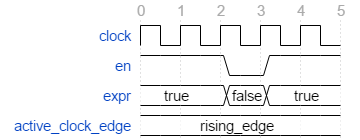`expr` is evaluated on every rising clock edge except for edge 3 where `en` is low. This means that the check will pass despite the false `expr` in the third clock cycle.

#### (True) Check (check and check_true)¶

Specific Parameter

Type

expr

boolean or std_logic

`check_true` is a more verbose version of `check` which emphasises that we’re expecting `expr` to be `true`/`1`/`H`. The extra verbosity is also present when the `result` function is used.

```check(false, result("for my data.");
```

will result in

```1000 ps - check - ERROR - Check failed for my data.
```

while

```check_true(false, result("for my data.");
```

will result in

```1000 ps - check - ERROR - True check failed for my data.
```

#### False Check (check_false)¶

Specific Parameter

Type

expr

boolean or std_logic

`check_false` passes when `expr` is `false`/`0`/`L`.

#### Implication Check (check_implication)¶

Specific Parameter

Type

antecedent_expr

boolean or std_logic

consequent_expr

boolean or std_logic

The unclocked subprograms use `boolean` parameters while the clocked procedures use `std_logic`.

`check_implication` checks logical implication and passes unless `antecedent_expr` is `true`/`1`/`H` and `consequent_expr` is `false`/`0`/`L`.

#### Not Unknown Check (check_not_unknown)¶

Specific Parameter

Type

expr

std_logic_vector or std_logic

`check_not_unknown` passes when `expr` contains none of the metavalues `U`, `X`, `Z`, `W`, or `-`.

#### Zero One-Hot Check (check_zero_one_hot)¶

Specific Parameter

Type

expr

std_logic_vector

`check_zero_one_hot` passes when `expr` contains none of the metavalues `U`, `X`, `Z`, `W`, or `-` and there are zero or one bit equal to `1` or `H` .

#### One-Hot Check (check_one_hot)¶

Specific Parameter

Type

expr

std_logic_vector

`check_one_hot` passes when `expr` contains none of the metavalues `U`, `X`, `Z`, `W`, or `-` and there is exactly one bit equal to `1` or `H` .

### Relation Checks¶

Relation checks are used to check whether or not a relation holds between two expressions, for example if `(a + b) = c`. They support the following six unclocked formats.

```procedure check_<name>(
[constant checker         : in  checker_t;]
[variable pass            : out boolean;]
<specific parameters>
constant msg             : in string := result;
constant level           : in log_level_t := null_log_level;
<preprocessor parameters>);
```
```impure function check_<name>(
[constant checker         : in  checker_t;]
<specific parameters>
constant msg             : in string := result;
constant level           : in log_level_t := null_log_level;
<preprocessor parameters>)
return boolean;
```

#### Equality Check (check_equal)¶

Specific Parameter

got

expected

The `got` and `expected` parameters can have the following combination of types

got

expected

unsigned

unsigned

natural

unsigned

unsigned

natural

natural

std_logic_vector

std_logic_vector

natural

std_logic_vector

std_logic_vector

std_logic_vector

unsigned

unsigned

std_logic_vector

signed

signed

integer

signed

signed

integer

integer

integer

std_logic

std_logic

boolean

std_logic

std_logic

boolean

boolean

boolean

time

time

string

string

Preprocessor Parameter

Type

Default Value

line_num

natural

0

file_name

string

“”

`check_equal` passes when `got` equals `expected`. When comparing `std_logic` values with `boolean` values `1` equals `true` and `0` equals `false`. Note that the `std_logic` don’t care (`-`) only equals itself. If you want an equality like `"0011" = "00--"` to pass you should use `check_relation` with the matching equality operator (`?=`) or `check_match` instead.

If a check fails you will get a context on the following format.

```Got <got value>. Expected <expected value>.
```

When you compare bit vectors, `integer` and `natural` type of values the error message will output the values on both formats. For example, here is a context when a `check_equal` between an `integer` and a `signed` value fails.

```Got 17 (0001_0001). Expected 0001_0000 (16).
```
##### Real value checks¶

For real values exact comparison is often not desired. Thus there is a `check_equal` variant taking a `max_diff` argument. The check fails if the absolute difference of the got and expected values are larger than `max_diff`.

```check_equal(0.1, 0.2, max_diff => 0.1); -- Passes
check_equal(0.1, 0.2, max_diff => 0.05); -- Fails
```
```Equality check passed - Got abs (0.1 - 0.2) <= 0.1.
Equality check failed - Got abs (0.1 - 0.2) > 0.05.
```

#### Relation Check (check_relation)¶

Specific Parameter

Type

expr

boolean, std_ulogic, or bit

Preprocessor Parameter

Type

Default Value

context_msg

string

“”

line_num

natural

0

file_name

string

“”

`expr` is intended to be a relational expression and three different types are supported. In case a matching relational operator is used the relation will return a `std_ulogic` or `bit` depending on the operands. All other relations will return a boolean.

`check_relation` passes when `expr` evaluates to `true` in the boolean case and to `1` in the `std_ulogic` and `bit` cases. This means that the `boolean` case behaves just like `check` and `check_true`. The additional value of this check comes when you enable the check preprocessor in your VUnit run script.

```ui = VUnit.from_argv()
ui.enable_check_preprocessing()
```

The check preprocessor scans your code for calls to `check_relation` and then parses `expr` as a VHDL relation. From that it will generate a context (context_msg parameter) describing how the relation failed. For example, the check

```check_relation(real_time_clock <= timeout, "Response too late");
```

will generate the following error message if it fails.

```1000 ps - check - ERROR - Response too late - Expected real_time_clock <= timeout. Left is 23:15:06. Right is 23:15:04.
```

This works for any type of relation between any types as long as the operator and the `to_string` function are defined for the types involved. In the example the operands are of a custom `clock_t` type for which both the `<=` operator and the `to_string` function have been defined.

Note that `context_msg` is the empty string by default so without the check preprocessor the error message will be just the `msg` provided by the user.

##### Relations with Side Effects¶

The left and right hand sides of the relation are evaluated twice, once when the relation is evaluated and once to create the error message so if you have a call like this

```check_relation(counter_to_verify = get_and_increment_reference_counter(increment_with => 3));
```

The reference counter will be incremented with 6 which is not what you expect by just looking at the code before the preprocessor has generated the `context_msg` which will be a string containing `to_string(get_and_increment_reference_counter(increment_with => 3))`.

Conclusion: Do not use impure functions in your expression. If you have a case like this you can do something like

```ref_cnt := get_and_increment_reference_counter(increment_with => 3);
check_relation(counter_to_verify = ref_cnt);
```

or since this is an equality relation, probably between standard countable types, use `check_equal` instead. `check_equal` has the left and right hand operands separated in the call itself so in that case there is no need for a second evaluation in order to create the error message.

##### Fooling the Parser¶

The check preprocessor has a simplified parser to determine what the relation operator in the expression is and what the left and right hand operands are. For example, it knows that this is an inequality since that is the only relational operator on the “top-level”.

```check_relation((a = b) /= (c = d));
```

It also knows that this isn’t a relation since there’s no relational operator on the top-level.

```check_relation((a = b) and c);
```

This will result in a syntax error from the check preprocessor

```SyntaxError: Failed to find relation in check_relation((a = b) and c)
```

However, its knowledge about precedence is limited to parenthesis so it will not understand that this identical expression isn’t a relation.

```check_relation(a = b and c);
```

If this logical expression returns false the check will generate an error message claiming that a relation failed and that `to_string(a)` was the left value and `to_string(b and c)` was the right value.

Conclusion: Use `check_relation` for relations as intended!

It should also be noted that the parser can handle that there are relational operators within the check call but outside of the `expr` parameter. For example, it won’t be fooled by the relational operators appearing within strings and comments of this call.

```check_relation(len("""Heart"" => <3") = -- The string contains <, so does
-- this comment
12, "Incorrect length of ""<3 string"".");
```

#### Match Check (check_match)¶

Specific Parameter

got

expected

The `got` and `expected` parameters can have the following combination of types

got

expected

unsigned

unsigned

std_logic_vector

std_logic_vector

signed

signed

std_logic

std_logic

Preprocessor Parameter

Type

Default Value

line_num

natural

0

file_name

string

“”

`check_match` passes when `got` equals `expected` but differs from `check_equal` in that a don’t care (`-`) bit equals anything.

### Sequence Checks¶

Sequence checks are checks that use several clock cycles to determine whether or not the desired property holds.

#### Stability Check (check_stable)¶

`check_stable` supports four different clocked formats. The `expr` parameter can be `std_logic` or `std_logic_vector` and the call can be made with or without the initial custom checker parameter.

```procedure check_stable(
[constant checker           : in checker_t;]
signal clock               : in std_logic;
signal en                  : in std_logic;
signal start_event         : in std_logic;
signal end_event           : in std_logic;
signal expr                : in std_logic or std_logic_vector;
constant msg               : in string      := result;
constant level             : in log_level_t := null_log_level;
constant active_clock_edge : in edge_t      := rising_edge;
constant allow_restart     : in boolean     := false;
constant line_num          : in natural     := 0;
constant file_name         : in string      := "");
```

`check_stable` passes if the `expr` parameter is stable in the window defined by the `start_event` and `end_event` parameters. The window starts at an active (according to `active_clock_edge`) and enabled (`en = '1'`) clock edge for which `start_event = '1'` and it ends at the next active and enabled clock edge for which `end_event = '1'`. `expr` is sampled for a reference value at the start event and is considered stable if it keeps that reference value at all enabled active clock edges within the window, including the clock edge for the end event. Bits within `expr` may change drive strength (between `'0'` and `'L'` or between `'1'` and `'H'`) and still be considered stable. Below is an example with two windows that will pass.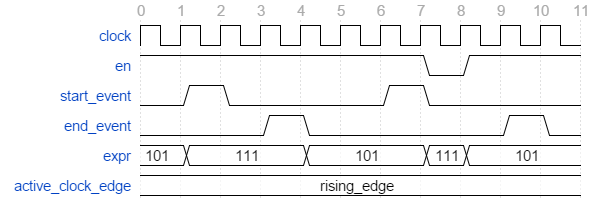Here are two examples of failing checks. Note that any unknown value (`U`, `X`, `Z`, `W`, or `-`) will cause the check to fail even if the unknown value is constant. The check will also fail if `start_event` or `end_event` in an active window has an unknown value.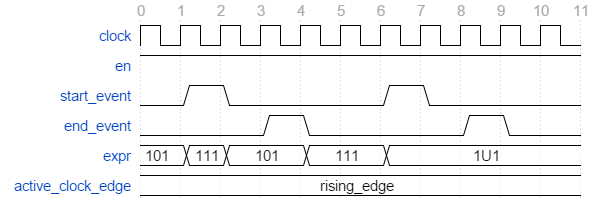`check_stable` can handle one clock cycle windows and back-to-back windows.

When `allow_restart` is `false` `check_stable` will ignore additional start events in the window. When `allow_restart` is `true` a new window is started if a new start event appears before the end event. The previous window is implicitly closed in the clock cycle before the new start event. An end event will still close the window if it appears before a second start event.

#### Next Check (check_next)¶

`check_next` supports two different formats. One with and one without the initial custom checker parameter.

```procedure check_next(
[constant checker           : in checker_t;]
signal clock                 : in    std_logic;
signal en                    : in    std_logic;
signal start_event           : in    std_logic;
signal expr                  : in    std_logic;
constant msg                 : in    string      := result;
constant num_cks             : in    natural     := 1;
constant allow_overlapping   : in    boolean     := true;
constant allow_missing_start : in    boolean     := true;
constant level               : in    log_level_t := null_log_level;
constant active_clock_edge   : in    edge_t      := rising_edge;
constant line_num            : in    natural     := 0;
```

`check_next` passes if `expr = '1'` `num_cks` active (according to `active_clock_edge`) and enabled (`en = '1'`) clock edges after a start event. The start event is defined by an active and enabled clock edge for which `start_event = '1'`. Below is an example of a passing check. The start event is sampled at clock edge two. `expr` is expected to be high four enabled clock edges after that which is at clock edge seven due to `en` being low at clock edge five.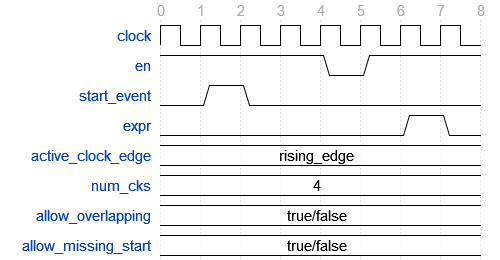When `allow_overlapping` is `true` `check_next` will allow a new start event before the check based on the previous start event has been completed. Here is an example with two overlapping and passing sequences.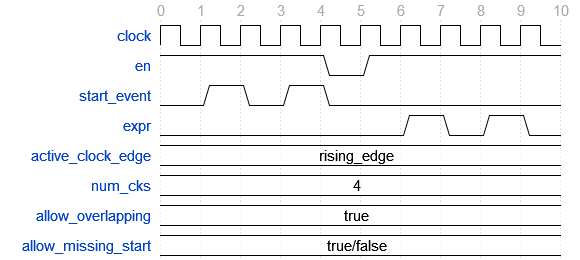In case `allow_overlapping` is `false` `check_next` will fail at the second start event

When `allow_missing_start` is `true` `check_next` will allow `expr = '1'` when there is no corresponding start event. When `allow_missing_start` is `false` such a situation will lead to a failure.

Any unknown value (`U`, `X`, `Z`, `W`, or `-`) on `start_event` will cause an error.

`check_next` will handle the weak values `L` and `H` in the same way as `0` and `1`, respectively.

#### Sequence Check (check_sequence)¶

`check_sequence` supports two different formats. One with and one without the initial custom checker parameter.

```procedure check_sequence(
[constant checker             : in checker_t;]
signal clock                 : in std_logic;
signal en                    : in std_logic;
signal event_sequence        : in std_logic_vector;
constant msg                 : in string          := result;
constant trigger_event       : in trigger_event_t := penultimate;
constant level               : in log_level_t     := null_log_level;
constant active_clock_edge   : in edge_t          := rising_edge;
constant line_num            : in natural         := 0;
constant file_name           : in string          := "");
```

`check_sequence` passes if a number of events, represented by the bits in the `event_sequence` parameter, are activated (bit = `'1'` or `'H'`) in sequence at consecutive active (according to `active_clock_edge`) and enabled (`en = '1'`) clock edges. `check_sequence` supports three different modes of operation as controlled by the `trigger_event` parameter:

• `first_pipe` - The sequence is started when the leftmost bit of `event_sequence` is activated. This will also trigger `check_sequence` to verify that the remaining bits are activated at the following active and enabled clock edges. `check_sequence` will also verify new sequences starting before the first is completed.

The figure below shows two overlapping sequences that pass.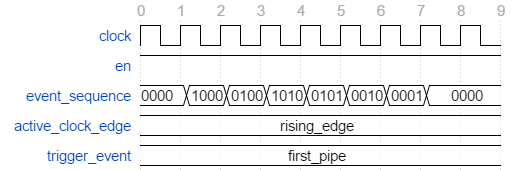In this example the sequence is started but not completed and the check fails.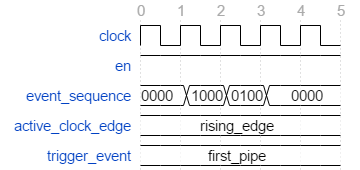• `first_no_pipe` - Same as `first_pipe` with the exception that only one sequence is verified at a time. New sequences starting before the previous is verified will be ignored.

In this example we have two sequences, the first is completed while the second is interrupted. However, since only one sequence is handled at a time the second is ignored and the check pass.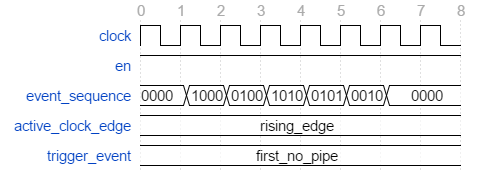• `penultimate` - The difference with the previous modes is that `check_sequence` only verifies the last event (the rightmost bit) when all the preceding events in the sequence have been activated. This means that a started sequence that is interrupted before the second to last bit is activated will pass. `check_sequence` will also verify new sequences starting before the first is completed.

The figure below shows two overlapping sequences which pass and then an early interrupted sequence that doesn’t cause a failure in this mode (which it did in the example for the `first_pipe` mode.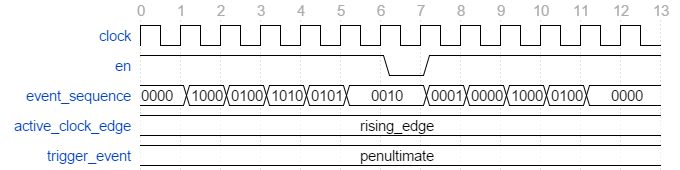In this example the sequence is interrupted after the second to last bit is activated and the check fails.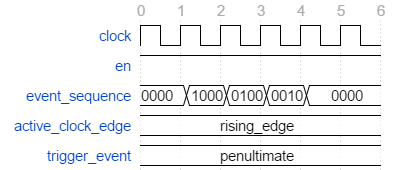Any unknown values (`U`, `X`, `Z`, `W`, or `-`) in `event_sequence` will lead to a an error. This is regardless of the mode of operation.

### Unconditional Checks¶

The check library has two unconditional checks, `check_passed` and `check_failed`, that contains no expression parameter to evaluate. They are used when the pass/fail status is already given by the program flow. For example,

```if <some condition> then
<do something>
check_passed;
else
<do something else>
check_failed("This was not expected");
end if;
```

With no `expr` parameter there are also fewer usable formats for these checkers.

```procedure check_passed(
[constant checker  : in checker_t;]
constant msg       : in string      := result(".");
constant line_num  : in natural     := 0;
constant file_name : in string      := "");
```
```procedure check_failed(
[constant checker   : in checker_t;]
constant msg       : in string      := result(".");
constant level     : in log_level_t := null_log_level;
constant line_num  : in natural     := 0;
constant file_name : in string      := "");
```